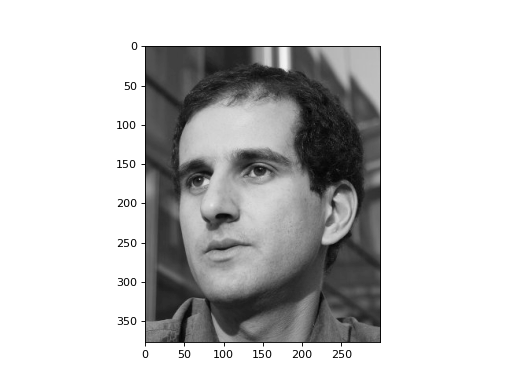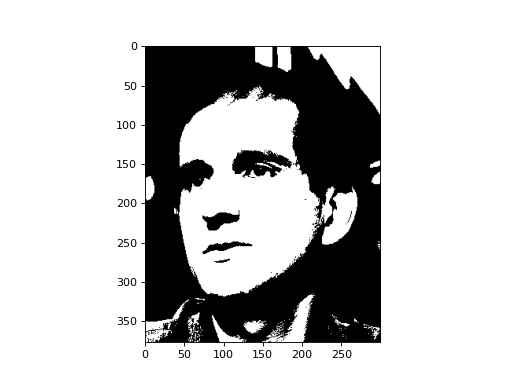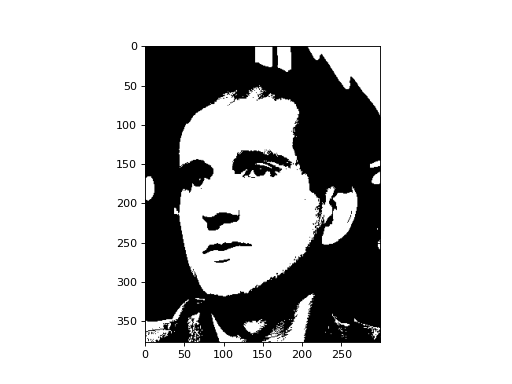# Thresholding¶

The example in this section is present in the source under `mahotas/demos/thresholding.py`.

We start with an image, a grey-scale image:

```import mahotas.demos
photo = photo.astype(np.uint8)
```

Before Oct 2013, the `mahotas.demos.load` function did not exist and you needed to specify the path explicitly:

```luispedro_image = '../../mahotas/demos/data/luispedro.jpg'
photo = mahotas.imread(luispedro_image, as_grey=True)
```

The reason we convert to `np.uint8` is because `as_grey` returns floating point images (there are good reasons for this and good reasons against it, since it’s easier to truncate than to go back, it returns `np.uint8`).

```import mahotas
import mahotas.demos
import numpy as np
from pylab import imshow, gray, show
from os import path

photo = mahotas.demos.load('luispedro', as_grey=True)
photo = photo.astype(np.uint8)

gray()
imshow(photo)
show()
```Thresholding functions have a trivial interface: they take an image and return a value. One of the most well-known thresholding methods is Otsu’s method:

```T_otsu = mahotas.otsu(photo)
print(T_otsu)
imshow(photo > T_otsu)
show()
```

prints `115`.

```import mahotas
import mahotas.demos
import numpy as np
from pylab import imshow, gray, show
from os import path

photo = mahotas.demos.load('luispedro', as_grey=True)
photo = photo.astype(np.uint8)

T_otsu = mahotas.otsu(photo)
print(T_otsu)
gray()
imshow(photo > T_otsu)
show()
```An alternative is the Riddler-Calvard method:

```T_rc = mahotas.rc(photo)
print(T_rc)
imshow(photo > T_rc)
show()
```

In this image, it prints almost the same as Otsu: `115.68`. The thresholded image is exactly the same:

```import mahotas
import mahotas.demos
import numpy as np
from pylab import imshow, gray, show
from os import path

photo = mahotas.demos.load('luispedro', as_grey=True)
photo = photo.astype(np.uint8)

T_rc = mahotas.rc(photo)
print(T_rc)
gray()
imshow(photo > T_rc)
show()
```See also the labeled documentation which can be very helpful in combination with thresholding.

## API Documentation¶

The `mahotas.thresholding` module contains the thresholding functions, but they are also available in the main `mahotas` namespace.

### Thresholding Module¶

Thresholding functions.

These functions return the numeric threshold. In order to obtain a thresholded image, you can do the following:

```T_otsu = mh.otsu(image)
binarized_image = (image > T_otsu)
```

Functions which have an `ignore_zeros` parameters will only consider non-zero pixels when computing the thresholding.

otsu(): Otsu method Riddler-Calvard’s method Bernsen thresholding Generalized Bernsen thresholding
`mahotas.thresholding.``otsu`(img, ignore_zeros=False)

Calculate a threshold according to the Otsu method.

Example:

```import mahotas as mh
import mahotas.demos

im = mahotas.demos.nuclear_image()
# im is stored as RGB, let's convert to single 2D format:
im = im.max(2)

#Now, we compute Otsu:
t = mh.otsu(im)

# finally, we use the value to form a binary image:
bin = (im > t)
```

See Wikipedia for details on methods: http://en.wikipedia.org/wiki/Otsu’s_method

Parameters: img : an image as a numpy array. This should be of an unsigned integer type. ignore_zeros : Boolean whether to ignore zero-valued pixels (default: False) T : integer the threshold
`mahotas.thresholding.``rc`(img, ignore_zeros=False)

Calculate a threshold according to the Riddler-Calvard method.

Example:

```import mahotas as mh
import mahotas.demos

im = mahotas.demos.nuclear_image()
# im is stored as RGB, let's convert to single 2D format:
im = im.max(2)

#Now, we compute a threshold:
t = mh.rc(im)

# finally, we use the value to form a binary image:
bin = (im > t)
```
Parameters: img : ndarray Image of any type ignore_zeros : boolean, optional Whether to ignore zero valued pixels (default: False) T : float threshold
`mahotas.thresholding.``soft_threshold`(f, tval)

Soft threshold function:

```                    ^
|           /
|          /
|         /
|        /
|       /
- - - - - - - - - - - - - - - - - ->
/      |
/       |
/        |
/         |
/          |
/           |
```
Parameters: f : ndarray tval : scalar thresholded : ndarray
`mahotas.thresholding.``bernsen`(f, radius, contrast_threshold, gthresh={128})

Bernsen local thresholding

Parameters: f : ndarray input image radius : integer radius of circle (to consider “local”) contrast_threshold : integer contrast threshold gthresh : numeric, optional global threshold to fall back in low contrast regions thresholded : binary ndarray

`gbernsen`
`mahotas.thresholding.``gbernsen`(f, se, contrast_threshold, gthresh)
`bernsen`